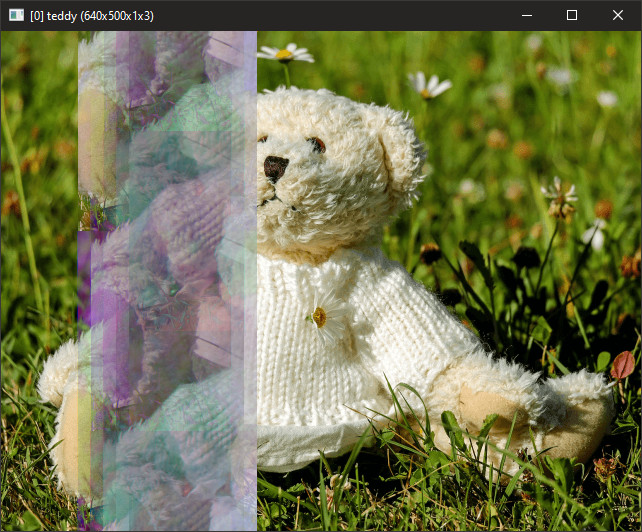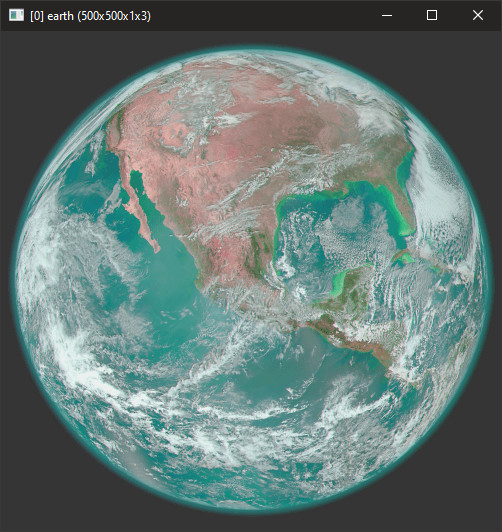Decided to move the subtopic found within ‘Glitch’ Art Filters - again into a whole thread to keep it focused. Inviting @Joan_Rake1

The point is to create a filter that can replace audacity databending.

The reason being:

1. It’s to explore algorithm used in sound and converting it into a g’mic-qt script.
2. It’s better as a collaborative project since there’s quite a lot of algorithms out there pertaining to sound manipulation.
3. Figure out some problems with the current code. (I know it’s for single image as this is experimental, and will do `repeat \$! l[\$>] endl done`.
4. Finally, figure out how to have several user-defined arguments as a vector and insert all of those arguments at once within the Insert Code block.
``````rep_auda cxzy
rep_auda:
('\$1')

l.
s x
remove_duplicates
a x
if w#-1!=4
error !dups?==F
fi
endl

4,1,1,1,"begin(numid_xyzc=[120,121,122,99];);
numid_xyzc[find(#-1,numid_xyzc[x],0,1)];"

img2text. ,

unpermute_string=\${}

rm[-2,-1]

permute \$1

whds={[w,h,d,s]}

unroll x

#Insert Code Here#
f int(x/(w/3))%2?j(i*(5*x/(w-1)-int(5*x/(w-1)))):i
#End#

r \$whds,-1

permute \$unpermute_string
``````

Allow me to explain what does this command does.

1. Create 4x1x1x1 image with chars converted to numbers. 120,121,122,99 respectively for ‘xyzc’.
2. On the local command, it checks if there are duplicates. I think it can be expanded to find invalid numbers, but I assume permute already does that even though I had not checked.
3. After the local part of command is finished, what this does is to find the unpermute string. It does this by inserting number to corresponding numbers respectively for ‘xyzc’.
4. img2text return numbers as series of characters.
5. unpermute_string=\${} insert the returned characters.
6. Then once the strings are checked out, those images that are numberical representation of characters are no longer needed.
7. permute swaps x,y,z,c id of image.
8. unroll converts into 1D image. This makes it possible to stimulate audacity filters.
9. This is where the user-defined processes go in.
10. Then r \$whds,-1 rolls it back basically.
11. Finally, permute using the reverse-permute string.

The one problem: What if I wanted to define 3 variables instead, and leave 1 variable as appended and is it really necessary? I know that for xyzc case, you can split into 4 and append channel together after you unrolled.

The rest, I will try to explore sound algorithm to the best of my ability. Others are welcomed to add code used for 1D processing.

`rep_linear_echo: repeat \$1 f lerp(j((\$2%*w)),i,x/\$3-int(x/\$3)) done`

Example:

`rep_washy_waves: f j(tan(x/(w/10))*(w/100)*.002*sin(x/10))`

``````rep_crop_and_replace:
+crop 10%,20%
f.. x>(.5*(w-1))&&x<=(.65*(w-1))?i(#-1,50+x%(w#-1-1)):i
rm.
``````
``````rep_stretch_and_replace:
f I(x/\$1,0,0,\$2,\$3)
``````
`````` sx={\$1%*w}
ex={\$2%*w}
dx={\$3%*w}
dg=1
dc=.2
edc={1/\$4*\$dc}
repeat \$4
nsx={\$sx+\$dx}
nex={\$ex+\$dx}
+crop \$sx,\$ex
+crop \$nsx,\$nex
*. \$dg
f. "
a=i(#-2);b=i;
mh=a+b;
lerp(a,b,b/(mh?mh:1));
"
j .,\$nsx
rm[-2,-1]
sx={\$nsx}
ex={\$nex}
dg-=\$edc
done
``````

Preview:``````rep_fade:
ww={w-1}
ix={int(\$1%*\$ww)}
ex={int(\$2%*\$ww)}
+crop \$ix,\$ex
l.
s x,\$3
ww={w-1}
if \$4 f i*(1-(x/\$ww)*.5)
else f i*x/\$ww*.5
fi
a x
endl
j.. .,\$ix
rm.
``````
``````rep_shuffle:
ww={w-1}
pxa={\$1%*\$ww}
pxb={\$2%*\$ww}
+crop \$pxa,\$pxb
ww={w-1}
l.
repeat \$3
m1={int(u(0,1)*\$ww)}
m2={int(u(0,1)*\$ww)}
m={min(\$m1,\$m2)},{max(\$m1,\$m2)}
n1={int(u(0,1)*\$ww)}
n2={int(u(0,1)*\$ww)}
n={min(\$m1,\$m2)},{max(\$m1,\$m2)}
+crop \$m
+crop \$n
j .,{min(\$m1,\$m2)}
j ..,{min(\$n1,\$n2)}
rm[-2,-1]
done
endl
j.. .,\$pxa
rm.
````````````rep_repeat_part:
+crop 5%,10%
+crop 12%,52%
f. i(#-2,x,0,0,0,1,2)
j .,{15%*(w-1)}
rm[-2,-1]
``````
``````rep_seawave_distort:
rw={(w-1)*50/100}
off={(w-1)*-.05/100}
f j(1-abs(((sqrt(1-((x%\$rw-\$rw)/\$rw)^2))-.5))^5*\$off,0,0,0,0,2)
``````
``````_iain_low_pass_filter:
if \$1>0 fill >i*\$1+j(-1)*(1-\$1)
else fill >i*abs(\$1)-j(-1)*(1-abs(\$1))
fi
``````1 Like

I wonder if you are over-complicating the problem. What dimensions would your inputs and outputs have? Why not process them as they are (besides the decoding and encoding stages)?

Same dimensions. The point is to make a filter that enables audacity-style databending, and that does not change dimension. Audio files are essentially 1d.

If we were in 1d space, the dimensions would be w,1,1,1 or 1,h,1,1. What are the other 10 steps for?

Ok, the first step is used to create of string \$1 which is used as a reference for unpermute string. The 2 is to check invalid inputs like xyxc which has two x in it. And 3 creates a image which are numbers that are represented as unpermute string. 4, and 5 finalize on that part by setting the image value converted to characters into unpermute_string. The rest is for audio-style processing. I’m not sure how I can explain better. If you try the code yourself, and change the between #Insert Code Here#, and #End, you may understand.

Here’s a test code:

``````rep_auda cxyz
``````

Oh, I think I get it now. We aren’t processing 1d data are we? We are making rolled oats, adding flavours and then reconstituting them.

Feast your eyes on these: https://www.musicdsp.org/en/latest/

Take this ladder filter for example: https://www.musicdsp.org/en/latest/Filters/253-perfect-lp4-filter.html

It might be possible to make a ladder filter of any integer order because of its iterative structure. I’m not sure if it’s possible to create an arbitrary integer number of variables and assign values to them in the parser though. It might even be possible to do something that isn’t just integer-order…

1 Like

That’s a great find, I wasn’t finding anything that close to legible as in easy to understand or at least close to there. I personally don’t understand it very well though I did managed to replicate some audacity functions at a elemental level, and echo is very much similar at a basic level. I’ll have a look at those in hope of finding something that’s easy to translate.

I found this. That seem very legible and understandable.

DSP in general is very tough, almost like magic. In music technology I see people doing some crazy things but a lot of the source code is closed and going through textbooks of material (mostly hard maths) is painfully slow. I’m still trying to find a way to convert a low pass like that into a high pass… Maybe this might help. https://www.musicdsp.org/en/latest/Filters/117-one-pole-one-zero-lp-hp.html

Soft saturation is really easy to implement but we don’t really need it - it’s a contrast enhancement. It’s a specific kind of waveshaper, and we already have that thanks to the curves graphs. A compressor isn’t as easy to make but it would have a very unusual effect.

We should note that we’ve got an advantage that musicians don’t: our signals aren’t live and we already have all the data we need. We don’t have to care about latency, so we can take samples anywhere within the image.

You’re right I didn’t need to implement it. Though maybe it would be more useful to specify within specific areas. I added `rep_fade`.

EDIT: Added `rep_shuffle`

It doesn’t check. As there aren’t many cases, you could cover all of them in your conditional.

I have an audio background so a lot of my thinking about image processing is translated into audio terms in my head.

There are two main differences between images and audio (besides the 1D/2D thing).:

1. Images have all positive values and audio has negative and positive values. Audio has rapid fluctuations from positive to negative, which is quite different to image data which, even if it fluctuates, tends to be smoother.

2. Audio processing generally is one sample at a time, with no knowledge of future samples. Images have all the pixels available to you at once.

IIRC A simple low pass filter in audio is:

(current sample x F) + (previous sample x (1-F) )

A high pass filter is:

(current sample x F) - (previous sample x (1-F) )

where F determines the cut-off frequency

So, for this filter to be accurate, I would need to find a way to convert the unrolled data in a way that audacity sees the sound. Yeah, let’s not bother figuring that out.

That’s quite what @Joan_Rake1 has mentioned.

Thanks for the low/high pass filter though I’m not sure about what is cut-off frequency or even stimulate it. That’s part of the reason that this filter would be a collaborative project since the number of audio filters is huge, and it can be difficult to understand the algorithm especially for me with no background in coding for audio processing.

Cut-off frequency relates approximately to blur radius for a low pass filter

``````#@gui low_pass_filter: low_pass_filter,low_pass_filter(0)
#@gui : cut-off = float (0.1,0,1)

low_pass_filter:

-fill >i*\$1+j(-1)*(1-\$1)``````
1 Like

@Iain I do understand what you are saying, as I have a long music background, in school and community. But I am a visual and auditory learner (mostly the latter). Could you provide a numerical and audio example? That would make it crystal clear. Thanks!

Not sure if I can give you an audio example easily, but numerically I will give a go.

If we process the pixels (or samples, but I will refer to pixels) from left to right with a low pass filter as I described with F=0.9

``````pixel number 1 (n1)  = n1 * 0.9 + n0 * 0.1
n2 = n2 * 0.9 + n1 * 0.1
n3 = n3 * 0.9 + n2 * 0.1
``````

etc…

but the effects of previous calculations accumulate so if effectively becomes:

``````n1  = n1 * 0.9
n2 = n2 * 0.9 + (n1 * 0.9) * 0.1
n3 = n3 * 0.9 + (n2 * 0.9 + (n1 * 0.9) * 0.1) * 0.1
n4 = n4 * 0.9 + ((n2 * 0.9 + (n1 * 0.9) * 0.1)* 0.1) * 0.1
``````

or (if I have done my maths right)

``````n1 = n1 * 0.9
n2 = n2 * 0.9 + n1 * 0.09
n3 = n3 * 0.9 + n2 * 0.09 + n1 * 0.009
n4 = n4 * 0.9 + n3 * 0.09 + n2 * 0.009 + n1 * 0.0009
``````

So each pixel is the average of all the preceding pixels weighted by distance from the current pixel.

By adjusting the proportion of current and previous samples in the calculation (F) you can determine how much effect distant pixels have on the current pixel. Similar to a Gaussian blur radius.

High frequencies in images is related to what photographers call sharpness. That is, difference in adjacent pixels. Mid frequencies are related to ‘local contrast’ that is a difference in pixel values over several pixels.

A small blur radius reduces high frequencies/sharpness, and larger blur radius affects mid-frequencies/local-contrast. So cut-off frequency is comparable to blur radius.

1 Like

I had tested it, it appears that lowpass/highpass filter either turns the image gray. Adding cut 0,255 as post-process step seem to lead to more interesting result.

Also, about/just did added the code into OP. And sample picture there too.

Your code is missing the ‘>’ at the begging which means that each pixel is processed sequentially (like audio).

A high pass filter will produce negative values, so I would add 128 after doing it to display the result.

1 Like